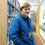# Recurring decimals as fractions

Earlier I solved this question and it got me thinking.

$\text{Why do recurring decimals have a fractional equivalent?}$

So I decided to work it out, this is what I got.

Let $b$ be a number with $n$ digits, it can have both leading and trailing zeros.

Every recurring decimal can now be represented by the decimal : $0.bbb\ldots$

To represent this as a fraction we need to see it as an infinite sum.

$0.bbb\ldots = \frac{b}{10^{n}} + \frac{b}{10^{2n}} + \frac{b}{10^{3n}} + \ldots$

Now that we have it as an infinite sum we can use the geometric series formula where

$a = \frac{b}{10^{n}}, r = \frac{1}{10^{n}}$

$\sum\limits_{x = 0}^{\infty} ar^{x} = \frac{a}{1 - r}$

Hence

$\sum\limits_{x = 0}^{\infty}\frac{b}{10^{n(x + 1)}} = \frac{\frac{b}{10^{n}}}{1 - \frac{1}{10^{n}}}$

$\large\frac{\frac{b}{10^{n}}}{1 - \frac{1}{10^{n}}} = \frac{\frac{b}{10^{n}}}{\frac{10^{n} - 1}{10^{n}}} = \frac{b}{10^{n}} \cdot \frac{10^{n}}{10^{n} - 1} = \frac{b}{10^{n} - 1}$

So that means that :

$0.bbb \ldots = \frac{b}{10^{n} - 1}$

But what if there's some decimal places in front of the recurring part?

Let $c$ be a number with $n_1$ digits, it can also have leading and trailing zeros.

The decimal is now : $0.cbbb \ldots$

Now we have to account for the decimal places between the decimal point and the recurring decimal. This is easy to do since we already have the equation for recurring decimals. The solution is simple - divide by $10$ to the power of the number of places ($n_1$).

$0.cbbb\ldots = \text{ ?} + \frac{b}{10^{n_1}(10^{n} - 1)}$

The $\text{?}$ represents the $c$ part of the decimal. It is simply the number $c$ divided by $10$ to the power of the number of decimal places ($n_1$) it takes up.

$0.cbbb\ldots = \frac{c}{10^{n_1}} + \frac{b}{10^{n_1}(10^{n} - 1)}$

Let's simplify it a bit.

$0.cbbb\ldots = \frac{b + c(10^{n} - 1)}{10^{n_1}(10^{n} - 1)}$

That's all for now, hope you found this note interesting.Note by Jack Rawlin
4 years, 7 months ago

This discussion board is a place to discuss our Daily Challenges and the math and science related to those challenges. Explanations are more than just a solution — they should explain the steps and thinking strategies that you used to obtain the solution. Comments should further the discussion of math and science.

When posting on Brilliant:

• Use the emojis to react to an explanation, whether you're congratulating a job well done , or just really confused .
• Ask specific questions about the challenge or the steps in somebody's explanation. Well-posed questions can add a lot to the discussion, but posting "I don't understand!" doesn't help anyone.
• Try to contribute something new to the discussion, whether it is an extension, generalization or other idea related to the challenge.

MarkdownAppears as
*italics* or _italics_ italics
**bold** or __bold__ bold
- bulleted- list
• bulleted
• list
1. numbered2. list
1. numbered
2. list
Note: you must add a full line of space before and after lists for them to show up correctly
paragraph 1paragraph 2

paragraph 1

paragraph 2

[example link](https://brilliant.org)example link
> This is a quote
This is a quote
    # I indented these lines
# 4 spaces, and now they show
# up as a code block.

print "hello world"
# I indented these lines
# 4 spaces, and now they show
# up as a code block.

print "hello world"
MathAppears as
Remember to wrap math in $$ ... $$ or $ ... $ to ensure proper formatting.
2 \times 3 $2 \times 3$
2^{34} $2^{34}$
a_{i-1} $a_{i-1}$
\frac{2}{3} $\frac{2}{3}$
\sqrt{2} $\sqrt{2}$
\sum_{i=1}^3 $\sum_{i=1}^3$
\sin \theta $\sin \theta$
\boxed{123} $\boxed{123}$

Sort by:

Great! Could you add this to the Converting Repeating Decimals into Fractions wiki? Thanks!

Staff - 4 years, 7 months ago